# Object detection and detection heads 2

Last updated on：a year ago

We are continuing to talk about regression/classification based framework.

# YOLO series

One-stage detectors based on global regression/classification, mapping from image pixels to bounding box coordinates and class probabilities, can reduce time expense.
The regression/classification based methods mainly include MultiBox, AttentionNet, G-CNN, YOLO, single shot MultiBox detection (SSD), YOLOv2, deconvolutional single shot detection (DSSD), and deeply supervised object detectors (DSOD).

## YOLO

You only look once (YOLO) is the first to frame object detection as a regression problem to spatially separated bounding boxes and associated class probabilities. Steps:

1. Resizes the input image to $448 \times 448$ 2) Runs a single convolutional network on the image, and 3) thresholds the resulting detections by the model’s confidence.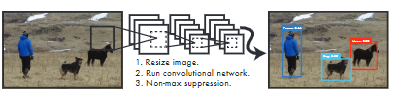YOLO divides the image into an $S \times S$ grid and for each grid cell predicts $B$ bounding boxes, confidence for those boxes, and $C$ class probabilities. These predictions are encoded as an $S \times S \times (B * 5 + C) tensor$.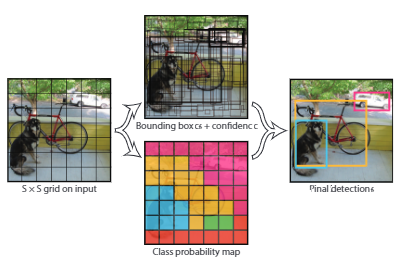YOLO algorithm
Codes:
Grid creating:
def create_grid(self, input_size):
w, h = input_size, input_size
# generate grid cells
ws, hs = w // self.stride, h // self.stride
grid_y, grid_x = torch.meshgrid([torch.arange(hs), torch.arange(ws)])
grid_xy = torch.stack([grid_x, grid_y], dim=-1).float()
grid_xy = grid_xy.view(1, hs*ws, 1, 2).to(self.device)

# generate anchor_wh tensor
anchor_wh = self.anchor_size.repeat(hs*ws, 1, 1).unsqueeze(0).to(self.device)

return grid_xy, anchor_wh
Decode boxes (from coordinates to corners)
def decode_xywh(self, txtytwth_pred):
"""
Input: \n
txtytwth_pred : [B, H*W, anchor_n, 4] \n
Output: \n
xywh_pred : [B, H*W*anchor_n, 4] \n
"""
B, HW, ab_n, _ = txtytwth_pred.size()
# b_x = sigmoid(tx) + gride_x
# b_y = sigmoid(ty) + gride_y
xy_pred = torch.sigmoid(txtytwth_pred[..., :2]) + self.grid_cell
# b_w = anchor_w * exp(tw)
# b_h = anchor_h * exp(th)
wh_pred = torch.exp(txtytwth_pred[..., 2:]) * self.all_anchor_wh
# [B, H*W, anchor_n, 4] -> [B, H*W*anchor_n, 4]
xywh_pred = torch.cat([xy_pred, wh_pred], -1).view(B, -1, 4) * self.stride

return xywh_pred

def decode_boxes(self, txtytwth_pred):
"""
Input: \n
txtytwth_pred : [B, H*W, anchor_n, 4] \n
Output: \n
x1y1x2y2_pred : [B, H*W*anchor_n, 4] \n
"""
# txtytwth -> cxcywh
xywh_pred = self.decode_xywh(txtytwth_pred)

# cxcywh -> x1y1x2y2
x1y1x2y2_pred = torch.zeros_like(xywh_pred)
x1y1_pred = xywh_pred[..., :2] - xywh_pred[..., 2:] * 0.5
x2y2_pred = xywh_pred[..., :2] + xywh_pred[..., 2:] * 0.5
x1y1x2y2_pred = torch.cat([x1y1_pred, x2y2_pred], dim=-1)

return x1y1x2y2_pred

## YOLOv2

You only look once version 2 (YOLOv2) uses k-means clustering on the dimensions of bounding boxes to get good priors. It predicts the width and height of the box as offsets from clustering centroids, and then the the box’s centre coordinates relative to the filter application’s location using a sigmoid function.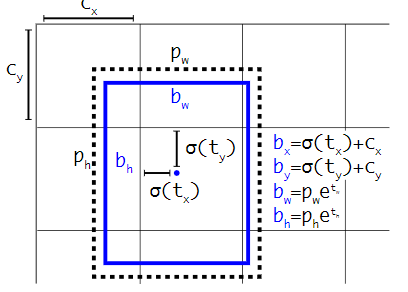Compared to YOLO, YOLOv2 uses batch normalisation, a higher resolution classifier, convolutional with anchor boxes, dimension cluster, direct location prediction, and multi-scale training.

## YOLOv3

You only look once version 3 (YOLOv3) makes increment in backbone and bounding box prediction.

def create_grid(self, input_size):
total_grid_xy = []
total_stride = []
total_anchor_wh = []
w, h = input_size, input_size
for ind, s in enumerate(self.stride):
# generate grid cells
ws, hs = w // s, h // s
grid_y, grid_x = torch.meshgrid([torch.arange(hs), torch.arange(ws)])
grid_xy = torch.stack([grid_x, grid_y], dim=-1).float()
grid_xy = grid_xy.view(1, hs*ws, 1, 2)

# generate stride tensor
stride_tensor = torch.ones([1, hs*ws, self.num_anchors, 2]) * s

# generate anchor_wh tensor
anchor_wh = self.anchor_size[ind].repeat(hs*ws, 1, 1)

total_grid_xy.append(grid_xy)
total_stride.append(stride_tensor)
total_anchor_wh.append(anchor_wh)

total_grid_xy = torch.cat(total_grid_xy, dim=1).to(self.device)
total_stride = torch.cat(total_stride, dim=1).to(self.device)
total_anchor_wh = torch.cat(total_anchor_wh, dim=0).to(self.device).unsqueeze(0)

return total_grid_xy, total_stride, total_anchor_wh

## YOLOv4

You only look once version 4 (YOLOv4) consists of YOLOv3 head, a modified darknet backbone, SPP neck and PAN neck. Most importantly, it becomes a two stages network.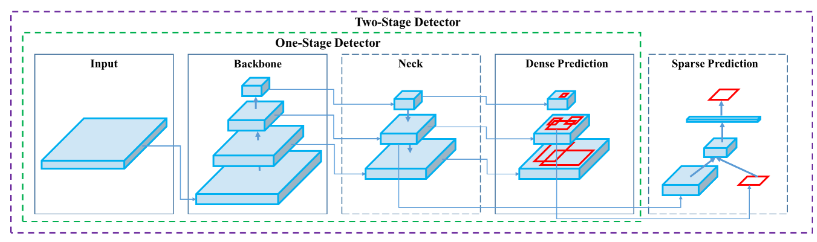Codes:
Spatial pyramid pooling (SPP) codes:

class SpatialPyramidPooling(nn.Module):
def __init__(self, feature_channels, pool_sizes=[5, 9, 13]):
super(SpatialPyramidPooling, self).__init__()

Conv(feature_channels[-1], feature_channels[-1] // 2, 1),
Conv(feature_channels[-1] // 2, feature_channels[-1], 3),
Conv(feature_channels[-1], feature_channels[-1] // 2, 1),
)

self.maxpools = nn.ModuleList(
[
nn.MaxPool2d(pool_size, 1, pool_size // 2)
for pool_size in pool_sizes
]
)
self.__initialize_weights()

def forward(self, x):
features = [maxpool(x) for maxpool in self.maxpools]
features = torch.cat([x] + features, dim=1)

return features

Modified path aggregation network (PAN) codes: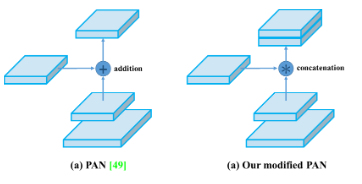class PANet(nn.Module):
def forward(self, features):
features = [
self.feature_transform3(features),
self.feature_transform4(features),
features,
]

downstream_feature5 = self.downstream_conv5(features)
downstream_feature4 = self.downstream_conv4(
torch.cat(
[features, self.resample5_4(downstream_feature5)], dim=1
)
)
downstream_feature3 = self.downstream_conv3(
torch.cat(
[features, self.resample4_3(downstream_feature4)], dim=1
)
)

upstream_feature4 = self.upstream_conv4(
torch.cat(
[self.resample3_4(downstream_feature3), downstream_feature4],
dim=1,
)
)
upstream_feature5 = self.upstream_conv5(
torch.cat(
[self.resample4_5(upstream_feature4), downstream_feature5],
dim=1,
)
)

return [downstream_feature3, upstream_feature4, upstream_feature5]

YOLOv4 codes:

class YOLOv4(nn.Module):
def forward(self, x):
atten = None
features = self.backbone(x)
if self.showatt:
features[-1], atten = self.attention(features[-1])
features[-1] = self.spp(features[-1])
features = self.panet(features)
predicts = self.predict_net(features)
return predicts, atten

## YOLOv5

No published paper. Codes in ultralytics/yolov5.

## YOLOv6

You only look once version 6 (YOLOv6) renovates network design, label assignment, loss function, data augmentation, industry-handy improvements, and quantisation and deployment.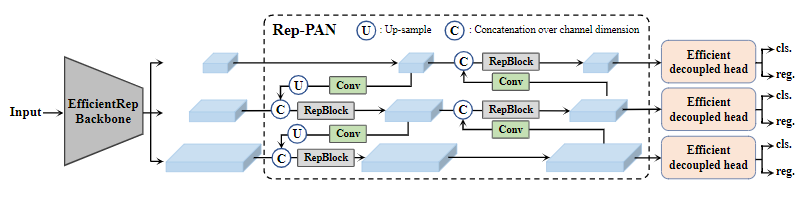RepBlock comprises a stack of RepVGG blocks with ReLU activations at training. During inference time, RepVGG block is converted to RepConv. CSPStackRep block includes three $1 \times 1$ convolutional layers and a stack of sub-blocks of double RepConvs following the ReLU activations with a residual connection.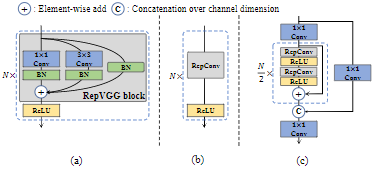RepPAN codes:

class RepPANNeck(nn.Module):
"""RepPANNeck Module
EfficientRep is the default backbone of this model.
RepPANNeck has the balance of feature fusion ability and hardware efficiency.
"""
def forward(self, input):

(x2, x1, x0) = input

fpn_out0 = self.reduce_layer0(x0)
upsample_feat0 = self.upsample0(fpn_out0)
if _QUANT:
upsample_feat0 = self.upsample_feat0_quant(upsample_feat0)
f_concat_layer0 = torch.cat([upsample_feat0, x1], 1)
f_out0 = self.Rep_p4(f_concat_layer0)

fpn_out1 = self.reduce_layer1(f_out0)
upsample_feat1 = self.upsample1(fpn_out1)
if _QUANT:
upsample_feat1 = self.upsample_feat1_quant(upsample_feat1)
f_concat_layer1 = torch.cat([upsample_feat1, x2], 1)
pan_out2 = self.Rep_p3(f_concat_layer1)

down_feat1 = self.downsample2(pan_out2)
p_concat_layer1 = torch.cat([down_feat1, fpn_out1], 1)
pan_out1 = self.Rep_n3(p_concat_layer1)

down_feat0 = self.downsample1(pan_out1)
p_concat_layer2 = torch.cat([down_feat0, fpn_out0], 1)
pan_out0 = self.Rep_n4(p_concat_layer2)

outputs = [pan_out2, pan_out1, pan_out0]

return outputs

## YOLOv7

You only look once version 7 (YOLOv7) also modifies the backbone, aggregation network, and concatenation.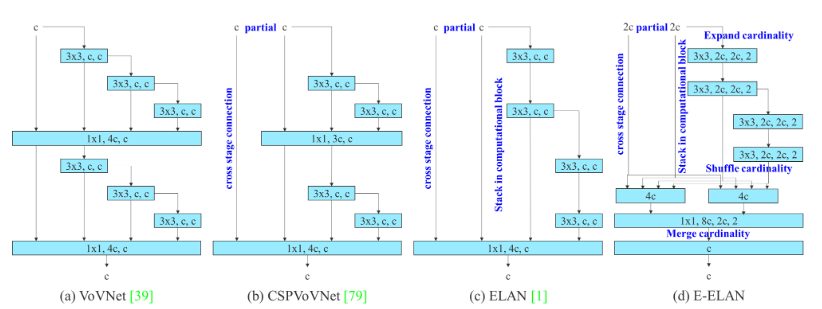# SSD series

We usually use SSD series to be the detection head for demonstrating our methods working better. Because SSD series outerperform YOLO series, though it is slower.

## SSD

Single shot multibox detector (SSD) discretises the output space of bounding boxes into a set of default boxes over different aspect ratios and scales per feature map location.
SSD model adds several feature layers to the end of a base network, which predicts the offsets to default boxes of different scales and aspect ratios and their associated confidences.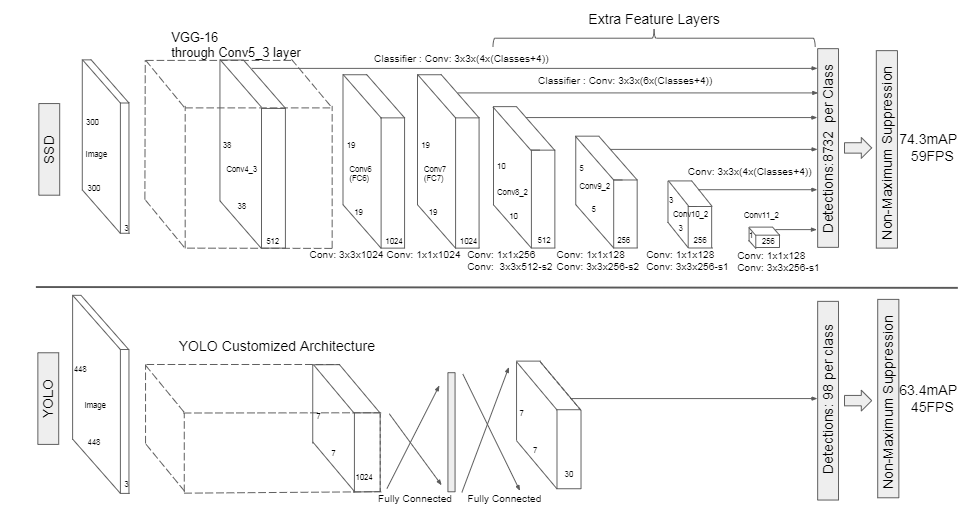SSD only needs an input image and ground truth boxes for each object during training. Different boxes of different aspect ratios at each location in several feature maps with different scales. For each default box, SSD predicts both shape offsets and the confidences for all object categories ($(c_1, c_2, …, c_p)$).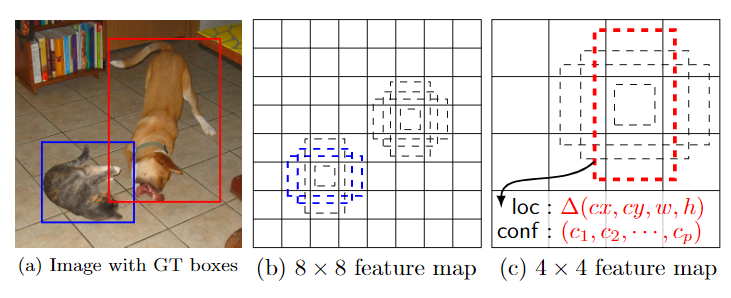The default boxes are matched to the ground truth boxes at the training time.
The overall objective loss function is a weighted sum of the localisation loss (loc) and the confidence loss (conf):
$$L(x, c, l, g) = \frac{1}{N} (L_{ \text{conf} } (x, c) + \alpha L_{ \text{loc} } (x, l, g) )$$
The localisation loss is a Smooth L1 loss between the predicted box ($l$) and the ground truth box ($g$) parameters.
$$L_{ \text{loc} } (x, l, g) = \sum_{i \in Pos\ m \in {cs, cy, w, h} }^N \sum x_{ij}^k \text{smooth}_{ \text{L1}} (l_i^m - \hat{g}_j^m)$$
Similar to Faster R-CNN, SSD regresses to offsets for the centre ($cx, cy$) of the default bounding box ($d$) and for its width ($w$) and height ($h$).
$$\hat{g}^{cx}_j = (g^{cx}_j - d^{cx}_i) / d^w_i$$

$$\hat{g}^{cy}_j = (g^{cy}_j - d^{cy}_i) / d^h_i$$

$$\hat{g}^{w}_j = \text{log} \frac{g^{w}_j}{d^w_i}$$

$$\hat{g}^{h}_j = \text{log} \frac{g^{h}_j}{d^h_i}$$

The confidence loss is the softmax loss over multiple classes of confidences ($c$), and the weight term $\alpha$ is set to 1 by cross validation.

$$L_{ \text {conf} } (x, c) = - \sum^N_{i \to Pos} x^p_{ij} \text{log} (\hat{c}^p_i) - \sum_{ i \to N eg} \text{log} (\hat{c}^0_i)$$

where,

$$\hat{c}^p_i = \frac{ \text{exp} (c^p_i) }{\sum_p \text{exp} (c^p_i)}$$

Codes:

class SingleShotMaskDetector(BaseDetHead):
def ssd_forward(
self,
end_points: Dict[str, Tensor],
device: Optional[torch.device] = torch.device("cpu"),
*args,
**kwargs
) -> Union[Tuple[Tensor, Tensor, Tensor], Tuple[Tensor, ...]]:

locations = []
confidences = []
anchors = []

x = end_points["os_{}".format(os)]
fm_h, fm_w = x.shape[2:]

locations.append(loc)
confidences.append(pred)

anchors_fm_ctr = self.AnchorBoxGenerator(
fm_height=fm_h, fm_width=fm_w, fm_output_stride=os, device=device
)
anchors.append(anchors_fm_ctr)

locations = torch.cat(locations, dim=1)
confidences = torch.cat(confidences, dim=1)

anchors = torch.cat(anchors, dim=0)
anchors = anchors.unsqueeze(dim=0)

return confidences, locations, anchors

def forward(
self, x: Union[Tensor, Dict]
) -> Union[Tuple[Tensor, ...], Tuple[Any, ...], Dict]:
if isinstance(x, Dict):
input_tensor = x["image"]
elif isinstance(x, Tensor):
input_tensor = x
else:
raise NotImplementedError(
"Input to SSD should be either a Tensor or a Dict of Tensors"
)

device = input_tensor.device
backbone_end_points: Dict = self.get_backbone_features(input_tensor)

if not isCoremlConversion(self.opt):
confidences, locations, anchors = self.ssd_forward(
end_points=backbone_end_points, device=device
)

output_dict = {"scores": confidences, "boxes": locations}

if not self.training:
# compute the detection results during evaluation
scores = nn.Softmax(dim=-1)(confidences)
boxes = self.match_prior.convert_to_boxes(
pred_locations=locations, anchors=anchors
)

detections = self.postprocess_detections(boxes=boxes, scores=scores)
output_dict["detections"] = detections

return output_dict
else:
return self.ssd_forward(end_points=backbone_end_points, is_prediction=False)

## SSDLite

Single Shot Detection (SSD) - Lite Version

## DSSD

Deconvolutional single shot detector (DSSD) augment SSD+Residual 101 with deconvolution layers to introduce additional large scale context in object detection and improve accuracy, especially for small objects.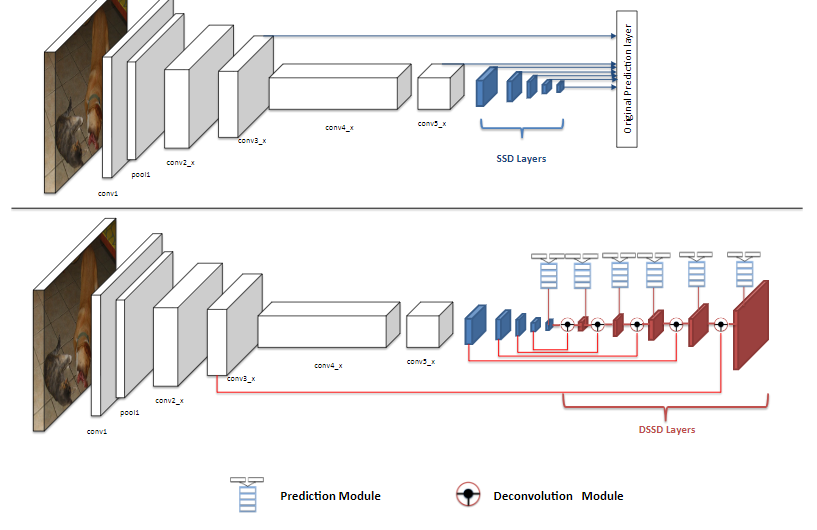DSSD deconvolutionnal layers: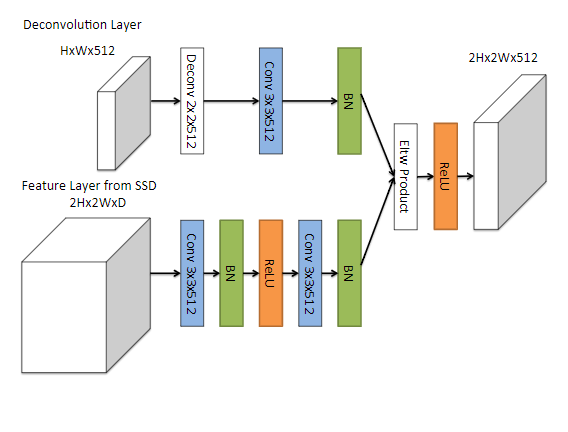Codes:

class DeconvolutionModule(nn.Module):
def __init__(self, cin_conv=1024, cin_deconv=512, cout=512, norm_layer=nn.BatchNorm2d, elementwise_type="sum", deconv_kernel_size=2, deconv_out_padding=0):
super(DeconvolutionModule, self).__init__()
self.conv_layer = nn.Sequential(
nn.Conv2d(cin_conv, cout, kernel_size=3, stride=1, padding=1, dilation=1, groups=1),
norm_layer(cout),
nn.ReLU(inplace=True),
nn.Conv2d(cout, cout, kernel_size=3, stride=1, padding=1, dilation=1, groups=1),
norm_layer(cout),
)

self.deconv_layer = nn.Sequential(
nn.Conv2d(cout, cout, kernel_size=3, stride=1, padding=1, dilation=1),
norm_layer(cout)
)

if elementwise_type in ["sum", "prod"]:
self.elementwise_type = elementwise_type
else:
raise RuntimeError("elementwise type incorrect!")
self.relu = nn.ReLU(inplace=True)

def forward(self, x_deconv, x_conv):
y_deconv = self.deconv_layer(x_deconv)
y_conv = self.conv_layer(x_conv)
if self.elementwise_type == "sum":
return self.relu(y_deconv + y_conv)
elif self.elementwise_type == "prod":
return self.relu(y_deconv + y_conv)

## DSOD

Deeply supervised object detector (DSOD) utilises dense connection for detection heads.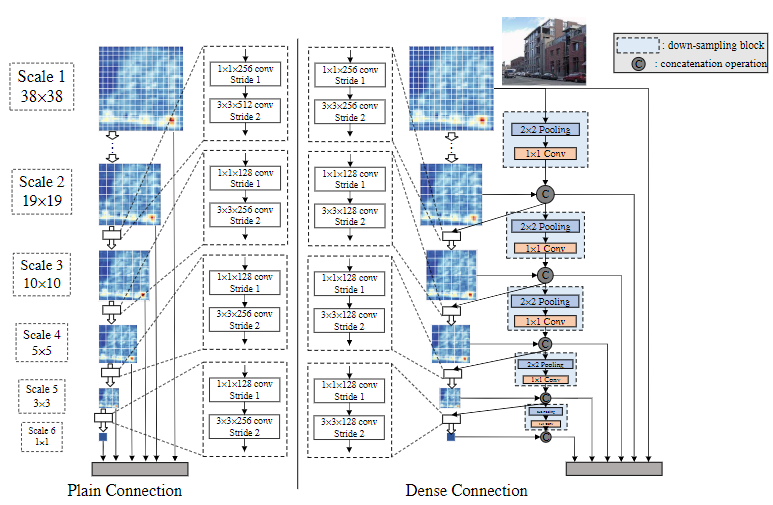## FSSD

Feature fusion single shot multibox detector (FSSD) introduce a lightweight feature fusion module that can improve the performance over SSD.
The proposed feature fusion and feature pyramid generation method. Features from different layers with different scales are concatenated together first and used to generate a series of pyramid features later.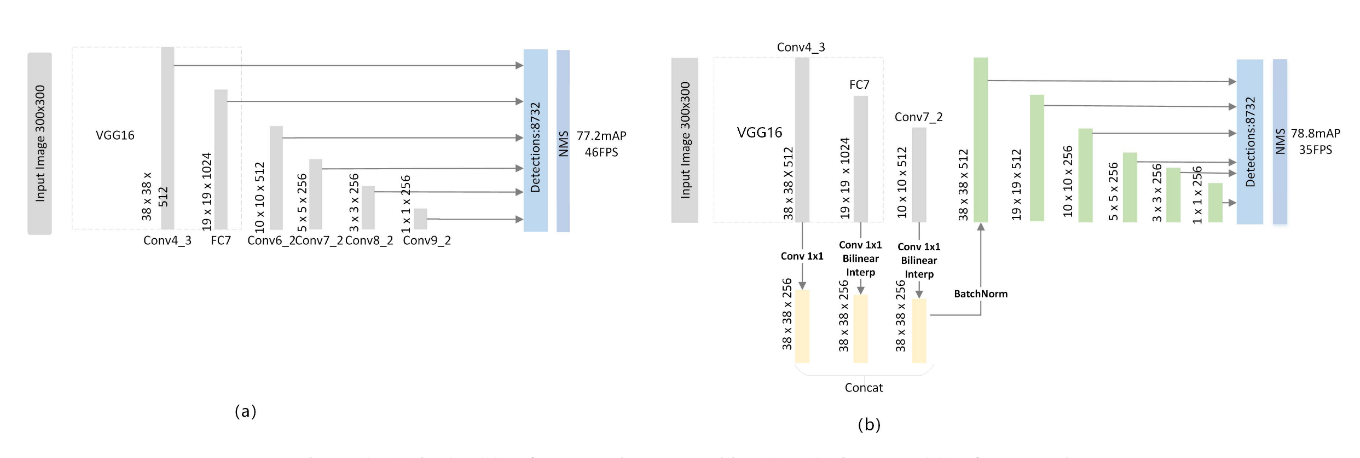# Reference

 Zhao, Z.Q., Zheng, P., Xu, S.T. and Wu, X., 2019. Object detection with deep learning: A review. IEEE transactions on neural networks and learning systems, 30(11), pp.3212-3232.
 Redmon, J., Divvala, S., Girshick, R. and Farhadi, A., 2016. You only look once: Unified, real-time object detection. In Proceedings of the IEEE conference on computer vision and pattern recognition (pp. 779-788).
 Redmon, J. and Farhadi, A., 2017. YOLO9000: better, faster, stronger. In Proceedings of the IEEE conference on computer vision and pattern recognition (pp. 7263-7271).
 yjh0410/yolov2-yolov3_PyTorch
 Redmon, J. and Farhadi, A., 2018. Yolov3: An incremental improvement. arXiv preprint arXiv:1804.02767.
 Bochkovskiy, A., Wang, C.Y. and Liao, H.Y.M., 2020. Yolov4: Optimal speed and accuracy of object detection. arXiv preprint arXiv:2004.10934.
 argusswift/YOLOv4-pytorch
 Li, C., Li, L., Jiang, H., Weng, K., Geng, Y., Li, L., Ke, Z., Li, Q., Cheng, M., Nie, W. and Li, Y., 2022. YOLOv6: A Single-Stage Object Detection Framework for Industrial Applications. arXiv preprint arXiv:2209.02976.
 meituan/YOLOv6
 Wang, C.Y., Bochkovskiy, A. and Liao, H.Y.M., 2022. YOLOv7: Trainable bag-of-freebies sets new state-of-the-art for real-time object detectors. arXiv preprint arXiv:2207.02696.
 Liu, W., Anguelov, D., Erhan, D., Szegedy, C., Reed, S., Fu, C.Y. and Berg, A.C., 2016, October. Ssd: Single shot multibox detector. In European conference on computer vision (pp. 21-37). Springer, Cham.
 Sandler, M., Howard, A., Zhu, M., Zhmoginov, A. and Chen, L.C., 2018. Mobilenetv2: Inverted residuals and linear bottlenecks. In Proceedings of the IEEE conference on computer vision and pattern recognition (pp. 4510-4520).
 Fu, C.Y., Liu, W., Ranga, A., Tyagi, A. and Berg, A.C., 2017. Dssd: Deconvolutional single shot detector. arXiv preprint arXiv:1701.06659.
 ZQPei/DSSD
 Shen, Z., Liu, Z., Li, J., Jiang, Y.G., Chen, Y. and Xue, X., 2017. Dsod: Learning deeply supervised object detectors from scratch. In Proceedings of the IEEE international conference on computer vision (pp. 1919-1927).
 Li, Z. and Zhou, F., 2017. FSSD: feature fusion single shot multibox detector. arXiv preprint arXiv:1712.00960.